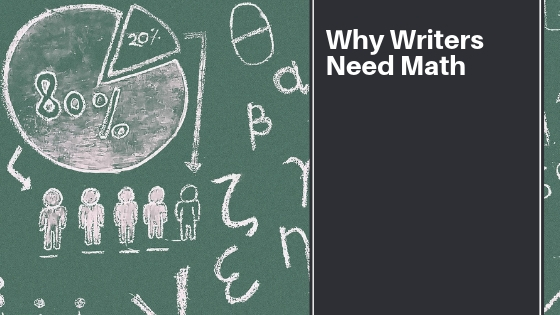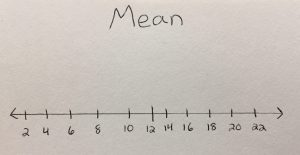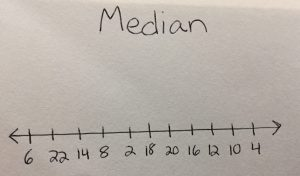# Why Writers Need to Learn Math#### “Mathematics is not about numbers, equations, computations, or algorithms: it’s about understanding.” – William Paul Thurston

I have never been too fond of math. Basic mathematics I can do with my eyes closed. Algebraic equations come easily to me until too many unknowns are thrown into the jumble. As far as geometry (and any math higher than that goes)–you may as well do the equation for me. So, when I had to learn math in journalism school–you can imagine how freaked out I was! But, the importance of math plays a vital role for writers and here’s why.

Percentage. Most writers that write for publications find themselves working in busy newsrooms. They write articles for newspapers, magazines or broadcast networks where percentages come into play. Here are some examples:

Unemployment is down 4.5%

11% of students favor the new cell phone policy

Half the battle of understanding percentages is learning how to calculate them. There are two different formulas: Remember, too, that percent means hundred. 5% stands for 5/100ths. Each of these formulas shows percent change.

Here’s the formula:
Old value – New Value ÷ Old Value × 100

Percentage increase: Let’s study the word problem below.

Jacob earns \$10 working at a grocery store. If his pay is increased to \$15, what is the increase?

First, we have to subtract the higher number from the lower number. 15 – 10 = 5.
Take this total and divide it by 10 (the original amount.)
It gives us a total of .5; now multiply by 100. The answer is 50%.

Percentage decrease: This time we’re going to flip things around. We take the lower number divided by the higher number. Let’s study the word problem below.

Samantha was earning \$13 / hour as a department manager. When she changed to a new job, now only earns \$9 / hour. What’s the percentage decrease?

First, we subtract 13 from 9, giving us a total of 4.
Next, we take 4 divided into 13.
When calculated, we get .30769231. Round up to .31 and multiply by 100, giving us a total of 31%.

Ages.
Another reason writers must learn math is so they can calculate ages. Writers of all different genres have to either write or fact-check ages in stories. Ages come into play when a journalist is writing a human interest story or a profile piece on a prominent person. Those that edit obituary pages also have to accurately check a person’s age each day. There’s a simple formula that you can use to make sure you are precise and correct. Here it is:

As an example, let’s say a person lived from June 1925 to May 2002.
If they died before their birthday, you would take 2001 – 1925. This tells you they are 76 years old.
Yet, if they died after their birthday, only then can you accurately take 2002 – 1925 — giving you 77 years old.

Statistics. Just the thought of it makes my head ache, but run with me here. It’s important that writers know the difference between mean, median, and mode.Mean is the average of a set of numbers. Use the image to the right as an example. First, you add up all the numbers within the sequence. This gives you a sum of 132. Next, divide that total by how many numbers there are in the set. There are eleven numbers in the set, so we take 132 ÷ 11. This equals a mean of 12.Next, we have the median. This is where you place the numbers of the sequence in order. The picture to the left is an example of how the numbers may appear unorganized. You would then organize them this way: {2, 4, 6, 8, 10, 12, 14, 16, 18, 20, 22}.

The following step is to locate the middle number and that is your median. In the mean set we have pictured above, the median is 12. But what if there was one more number on the end…24? In the case where you have two middle numbers, you add them together to find a total sum. Then, divide them to get an average. So, if this was the set: {2, 4, 6, 8, 10, 12, 14, 16, 18, 20, 22, 24} —

Add 12 + 14. This equals out to 26. Now, divide by 2. (There are 2 numbers.) Your median is 13.

And lastly, we have the mode. The mode is the number that appears the most frequently within a set. For instance: {5, 4, 3, 2, 4, 8, 9, 3, 4, 6}. In this set, 3 in the mode. All other numbers only appear once.

On another note, knowing how to read a graph is crucial. If you’re a news reporter, knowing the difference between a line graph (a graph which indicates change in variables by connection of a line), a bar graph (a graph that indicates data by height of bars represented by equal width) and a pie graph (a circular graph which divides data into sections). There may be other types of graphs, but these are the types of which I am aware as a writer. Learn them and understand their uses. You’re going to benefit from it.

If you need help brushing up on your math skills, I recommend hitting your local library. Check out used bookstores that sell math books. These are a great resource and you can math books of any level. Find books that have practice problems. Work through them slowly. Math is not easy, but once you know it, writing often becomes so much easier.

On a final note, I would recommend seeking out a math tutor. If math is not your strong suit, don’t be afraid to ask for help. Ask your friends or family if they know anyone that could help you work through the math problems. I’ve barely scratched the surface in the math writers need to know. There are such formulas as compound interest that also are important. Focus on the math that is most important to your industry. You got this!